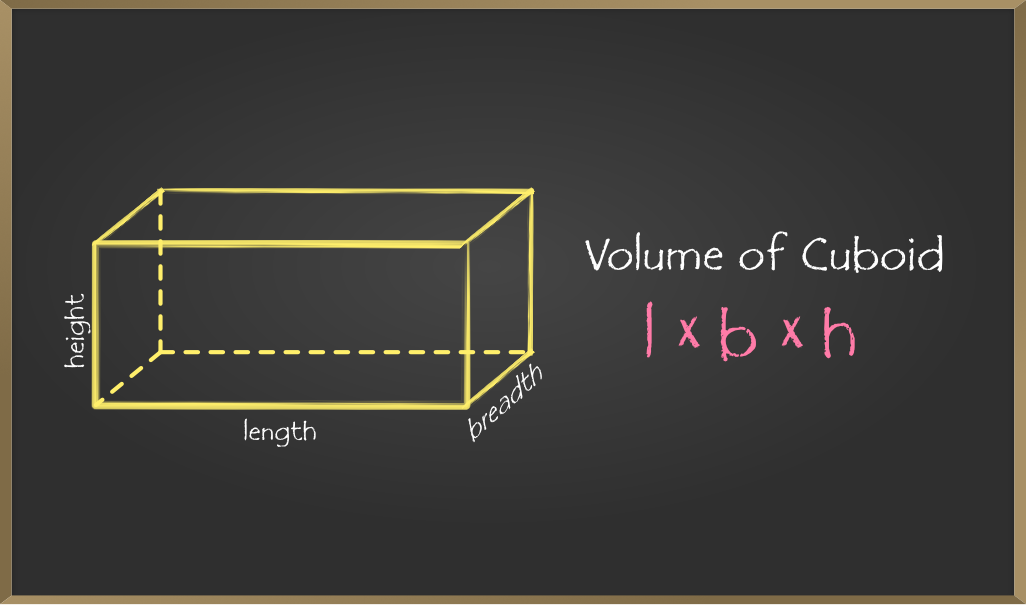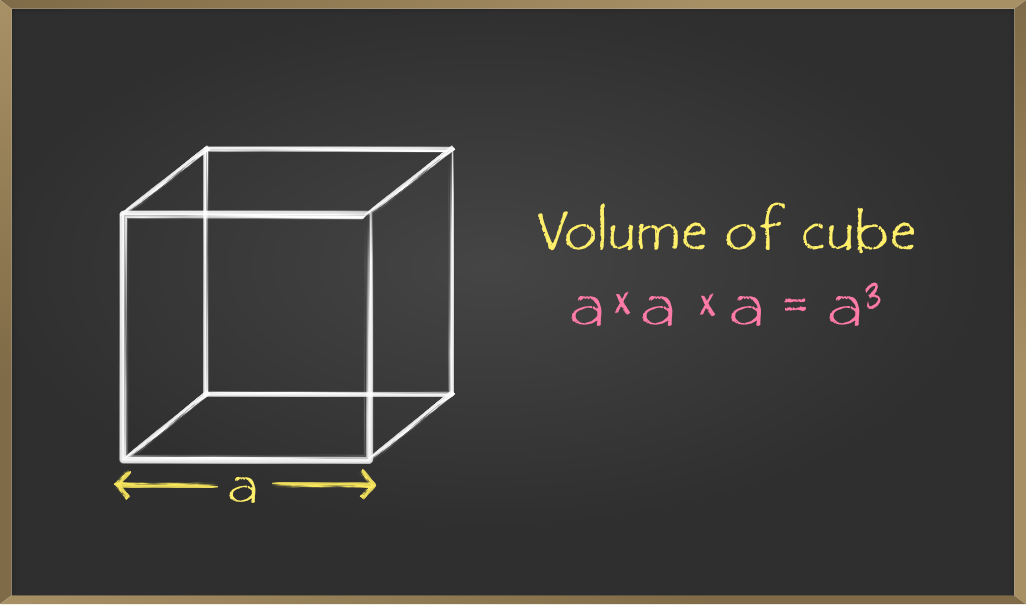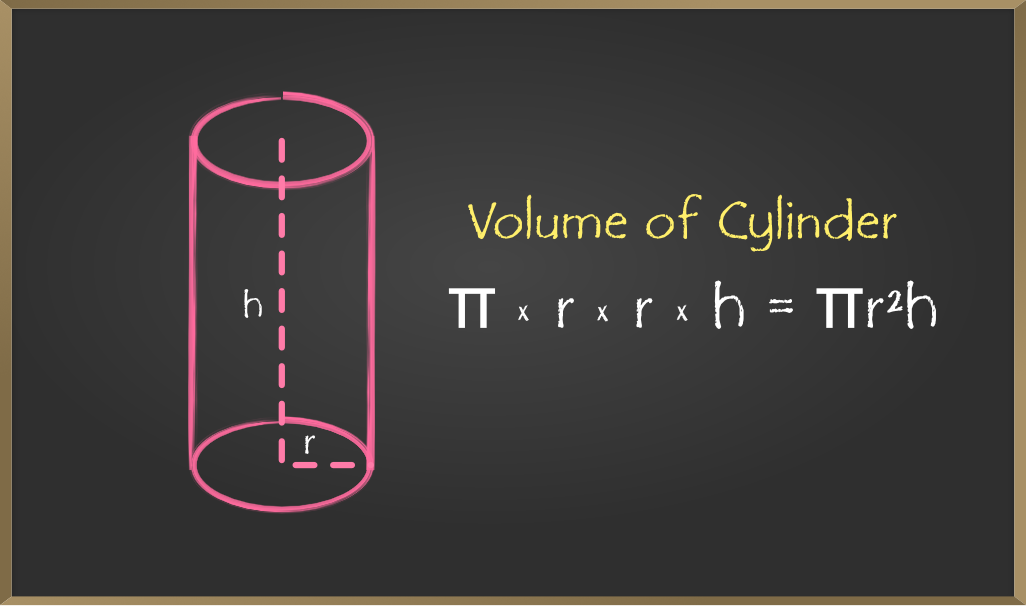GeeksforGeeks App
Open AppBrowser
Continue

# Mensuration – Volume of Cube, Cuboid, and Cylinder | Class 8 Maths

Mensuration the branch of mathematics which deals with the study of different geometrical shapes, their areas, and volume. It uses geometric calculations and algebraic equations to compute the measurement of various aspects of objects like Surface Area, Volume, etc.

Volume is the measurement of the amount of space inside a 3D object that can be filled. It can be the measurement of any solid objects like cube, square, cylinder, sphere, pyramid, etc. Volume of an object is usually measured using cubic units. By finding out the volume of an object it also helps us find the amount required to fill an object

The S.I unit of volume is m3. Other units are ml/L.

1m3 = 1 Litre

## Computing Volumes of 3D Objects

Till now you must have understood the meaning of volume, let’s move to finding the volume of various 3D geometrical figures like cube, cuboid, and cylinder.

### Finding Volume of a Cuboid

A cuboid is a three-dimensional structure having six rectangular faces. The volume of a cuboid is equal to the product of length, breadth, and height of the cuboid. In rectangular cuboid, all the angles are at right angles and the opposite faces of a cuboid are equal.

Let l is the length, b is the breadth and h is the height of the cuboid.Example 1: Calculate the volume of the cuboid where length, breadth, and height are 12, 8, and 4 meters respectively.

Solution: Given, Length=12m

Height = 4m

Applying the Formula,

Volume of cuboid = length x breadth x height

Volume of cuboid = 12 x 8 x 4 = 384m^3

Example 2: Calculate the breadth of the cuboid whose volume is given as 350-meter cube. And length and height are 7 and 5 meters respectively.

Solution: Given Volume of cuboid = length x breadth x height

350 = 7 x breadth x 5

### Volume of a Cube

Firstly the question arises what is cube? So, Cube is a  three-dimensional shape with equal width, height, and length measurements. Basically, a cuboid whose length, width, and height are equal is called a cube.

Since we know the volume of cuboid = length x breadth x height and the cube is a special case of cuboid where all length, breadth, and height are equal. Let’s assume length = breadth = height = aExample 1: Find the volume of a cube with sides of length 10 cm.

Solution: We know, V = (a ^ 3)

V = (10^3 cm)

So, Volume of cube = 300 centimeter cube

Example 2: Find the length of the sides of the cube whose volume is 343centimeter cube

Solution : Volume = side x side x side

343= (side)^3

7 cm = side

### Volume of Cylinder

A cylinder is a three-dimensional solid that contains two parallel bases connected by a curved surface. The bases are usually circular in shape. The perpendicular distance between the bases is denoted as the height “h” of the cylinder and “r” is the radius of the cylinder.Example 1: Calculate the volume of the cylindrical container having a radius of 4 cm and height of 35 cm.

(Take pi = 22/7)

Height = 35cm

Putting the values in the formulae,

Volume of cylinder = π x 4 x 4 x 35

= π x 560 (pi = 22/7)

= 1760 cm^3

Example 2: Calculate the radius of the cylinder  whose height is 21 cm and volume of the cylinder is 1100 centimeter cube?(Take pi = 22/7)

Solution: Volume of cylinder = πr2h  cubic units

1100 = 22/7 x r x r x 7

r = 7.07cm

My Personal Notes arrow_drop_up
Related Tutorials